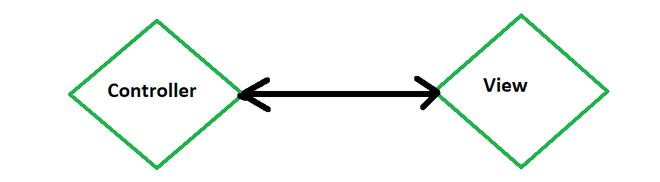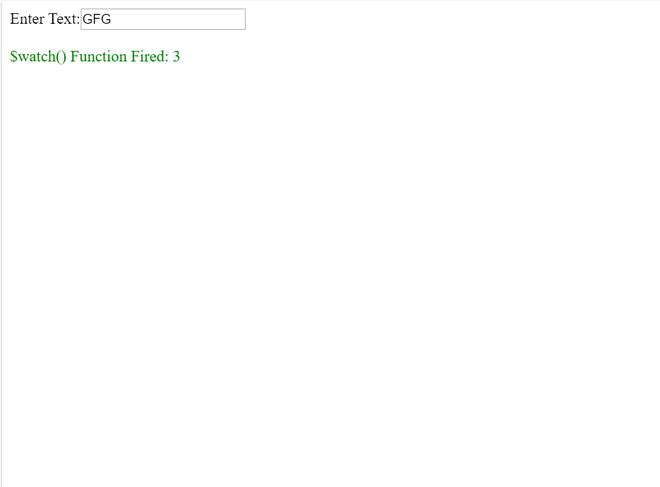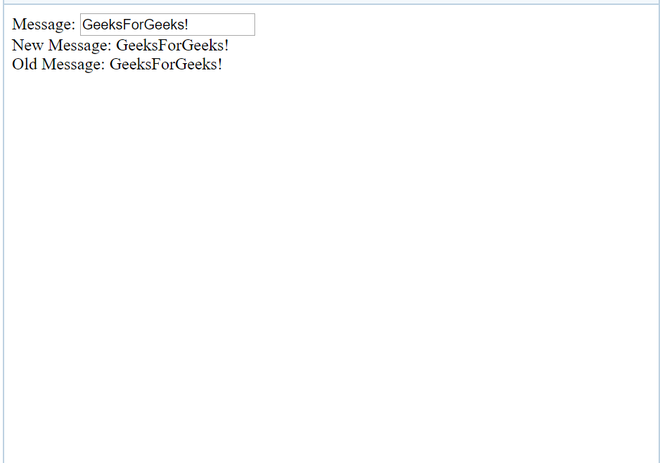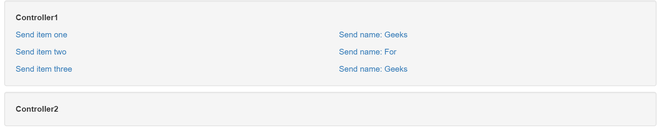# How to watch service variables ?

\$scope acts as a built-in object in AngularJs.

It consists of application data and methods. The \$scope is acts as a link between a controller and view (HTML).\$scope is used to transfer the data.

\$watch:

Scope in AngularJs object has \$watch event which comes into picture when the model property gets changed or altered.

When a data binding is created from some point of program in the view to a variable on the \$scope object, a watch is created internally in AngularJs.

The digest gets called multiple times, when we \$watch() a function.

Every time when we bind the UI, We insert \$watch in \$watch list.

```User: <input type="text" ng-model="user" />
```

Example:

 ` ` `<``html``> ` `    ``<``head``> ` `        ``<``title``> ` `            ``AngularJs \$watch() Function with GFG ` `        `` ` `        ``<``script` `src``= ` `"https://ajax.googleapis.com/ajax/libs/angularjs/1.4.8/angular.min.js"``> ` `      `` ` `        ``<``script` `type``=``"text/javascript"``> ` `            ``var app = angular.module("watchApp", []); ` `            ``app.controller("watchCtrl", function (\$scope) { ` `                ``\$scope.count = -1; ` `                ``\$scope.\$watch( ` `                  ``"txtval", function (newval, oldval) { ` `                    ``\$scope.count = \$scope.count + 1; ` `                ``}); ` `            ``}); ` `        `` ` `    `` ` `    ``<``body``> ` `        ``<``div` `ng-app``=``"watchApp"`  `             ``ng-controller``=``"watchCtrl"``> ` `            ``Enter Text:<``input` `type``=``"text"`  `                              ``ng-model``=``"txtval"` `/> ` `          ``<``br` `/> ` `            ``<``br` `/> ` `            ``<``span` `style``=``"color: Green;"``> ` `              ``\$watch() Function Fired: {{count}} ` `        `` ` `    `` ` ` `\$watch in AngularJs is a service.

It is used to keep the track of the changes on specific properties within a given scope. It is similar to an event listener. e changes are made in the value of specified variables.

When we register a watch we pass two functions as parameters to the \$watch() function.These functions are:

• A value function
• A listener function

Example:

```\$scope.\$watch(function() {}, //value function
function() {}  //listener function
);```

A watcher can change in responses on:

1. Timeouts
2. UI
3. Complex asynchronous computations performed by web workers
4. Ajax calls

Methods Used: \$scope.\$watchGroup

\$watchGroup() is a shortcut to set up watchers with the same callback, passing an array of watchExpressions.

\$scope.\$watchGroup([‘obj.a’, ‘obj.b.bb’, ‘letters’], function(newValues, oldValues, scope) {
//…
});

\$scope.\$watchCollection is a shortcut to watch arrays or objects. In arrays, the listener is called when any of the elements are replaced, deleted, or added.

The \$watch keeps track of the variable. This function takes two arguments:

1. new value

\$scope.\$watch(‘expression’, function (newvalue, oldvalue) { //expression parameter
//Code
});

2. old value

\$scope.\$watch(function () {}, function (newvalue, oldvalue) { //Function Parameter
// Code
});

Example:

 ` ` `<``html``> ` `<``head``> ` `    ``<``script` `src``= ` `"https://ajax.googleapis.com/ajax/libs/angularjs/1.3.16/angular.min.js"``> ` `   `` ` ` ` `<``body` `ng-app``=``"myNgApp"``> ` ` `  `    ``<``div` `ng-controller``=``"myController"``> ` `        ``Message: <``input` `type``=``"text"` `                        ``ng-model``=``"message"` `/>  ` `        ``<``br` `/> ` `        ``New Message: {{newMessage}} <``br` `/> ` `        ``Old Message: {{oldMessage}}  ` `    `` ` `    ``<``script``> ` `        ``var ngApp = angular.module('myNgApp', []); ` `     `  `        ``ngApp.controller('myController', function (\$scope) { ` `            ``\$scope.message = "GeeksForGeeks!"; ` ` `  `            ``\$scope.\$watch( ` `'message', function (newValue, oldValue) { ` `                ``\$scope.newMessage = newValue; ` `                ``\$scope.oldMessage = oldValue; ` `            ``}); ` `        ``}); ` `    `` ` ` ` ``Others:

\$digest()

We call the the \$digest() function when AngularJS thinks it is necessary.

For example, after a button click  handler is executed, or after an AJAX call returns.

\$apply():

The \$scope.\$apply() function takes a function as parameter which it is executed, and after that \$scope.\$digest() is called internally. That makes it helpful to all the watches are checked

```\$scope.\$apply(function() {
\$scope.data.myVar = "value";
});
```

Example of \$Watch:

 `<``div` `class``=``"container"` `data-ng-app``=``"app"``> ` `    ``<``div` `class``=``"well"` `data-ng-controller``=``"FooCtrl"``> ` `        ``<``p``><``strong``>Controller1 ` `        ``<``div` `class``=``"row"``> ` `            ``<``div` `class``=``"col-sm-6"``> ` `                ``<``p``><``a` `href``=``""`  `                      ``ng-click``= ` `   ``"setItems([ { name: 'I am single item' } ])"``> ` `                  ``Send item one ` `                ``<``p``><``a` `href``=``""`  `                      ``ng-click= ` `               ``"setItems([ { name: 'Item 1 of 2' }, ` `                  ``{ name: 'Item 2 of 2' } ])"> ` `                  ``Send item two ` `               `  `                ``<``p``><``a` `href``=``""` `                      ``ng-click= ` `                      ``"setItems([ { name: 'Item 1 of 3' }, ` `                  ``{ name: 'Item 2 of 3' }, ` `                  ``{ name: 'Item 3 of 3' } ])"> ` `                  ``Send item three ` `            `` ` `            ``<``div` `class``=``"col-sm-6"``> ` `                ``<``p``><``a` `href``=``""`  `                      ``ng-click``=``"setName('Sheldon')"``> ` `                  ``Send name: Geeks ` `                ``<``p``><``a` `href``=``""`  `                      ``ng-click``=``"setName('Leonard')"``> ` `                  ``Send name: For ` `                ``<``p``><``a` `href``=``""`  `                      ``ng-click``=``"setName('Penny')"``> ` `                  ``Send name: Geeks ` `            `` ` `        `` ` `    `` ` ` `  `    ``<``div` `class``=``"well"`  `         ``data-ng-controller``=``"BarCtrl"``> ` `        ``<``p``><``strong``>Controller2 ` `        ``<``p` `ng-if``=``"name"``>Name is: {{ name }} ` `        ``<``div` `ng-repeat``=``"item in items"``> ` `          ``{{ item.name }} ` `    `` ` ` `

 `var` `app = angular.module(``'app'``, []); ` ` `  `app.factory(``'PostmanService'``, ``function``() { ` `  ``var` `Postman = {}; ` `  ``Postman.set = ``function``(key, val) { ` `    ``Postman[key] = val; ` `  ``}; ` `  ``Postman.get = ``function``(key) { ` `    ``return` `Postman[key]; ` `  ``}; ` `  ``Postman.watch = ``function``(\$scope, key, onChange) { ` `    ``return` `\$scope.\$watch( ` `       `  `      ``function``() { ` `        ``return` `Postman.get(key); ` `      ``}, ` `       `  `      ``function``(newValue, oldValue) { ` `        ``if` `(newValue !== oldValue) { ` `          ``// Only update if the value changed ` `          ``\$scope[key] = newValue; ` `          ``// Run onChange if it is function ` `          ``if` `(angular.isFunction(onChange)) { ` `            ``onChange(newValue, oldValue); ` `          ``} ` `        ``} ` `      ``} ` `    ``); ` `  ``}; ` `  ``return` `Postman; ` `}); ` ` `  `app.controller(``'FooCtrl'``, [``'\$scope'``, ` `                           ``'PostmanService'``,  ` `             ``function``(\$scope, PostmanService) { ` `  ``\$scope.setItems = ``function``(items) { ` `    ``PostmanService.set(``'items'``, items); ` `  ``}; ` `  ``\$scope.setName = ``function``(name) { ` `    ``PostmanService.set(``'name'``, name); ` `  ``}; ` `}]); ` ` `  `app.controller(``'BarCtrl'``, [``'\$scope'``, ` `                           ``'PostmanService'``, ` `           ``function``(\$scope, PostmanService) { ` `  ``\$scope.items = []; ` `  ``\$scope.name = ``''``; ` `  ``PostmanService.watch(\$scope, ``'items'``); ` `  ``PostmanService.watch( ` `       ``\$scope,``'name'``, ``function``(newVal, oldVal) { ` `    ``alert(``'Hi, '` `+ newVal + ``'!'``); ` `  ``}); ` `}]);`

CSS:

 `.well { ` ` ``margin-top``: ``10px``; ` ` ``margin-bottom``: ``10px``; ` `} ` ` `  `p:last-child { ` ` ``margin-bottom``: ``0``; ` `} `My Personal Notes arrow_drop_upCheck out this Author's contributed articles.

If you like GeeksforGeeks and would like to contribute, you can also write an article using contribute.geeksforgeeks.org or mail your article to contribute@geeksforgeeks.org. See your article appearing on the GeeksforGeeks main page and help other Geeks.

Please Improve this article if you find anything incorrect by clicking on the "Improve Article" button below.

Article Tags :

Be the First to upvote.

Please write to us at contribute@geeksforgeeks.org to report any issue with the above content.